Intermediate Algebra 2e

# 9.6Graph Quadratic Functions Using Properties

Intermediate Algebra 2e9.6 Graph Quadratic Functions Using Properties

### Learning Objectives

By the end of this section, you will be able to:

• Recognize the graph of a quadratic function
• Find the axis of symmetry and vertex of a parabola
• Find the intercepts of a parabola
• Graph quadratic functions using properties
• Solve maximum and minimum applications

### Be Prepared 9.16

Before you get started, take this readiness quiz.

Graph the function $f(x)=x2f(x)=x2$ by plotting points.
If you missed this problem, review Example 3.54.

### Be Prepared 9.17

Solve: $2x2+3x−2=0.2x2+3x−2=0.$
If you missed this problem, review Example 6.45.

### Be Prepared 9.18

Evaluate $−b2a−b2a$ when a = 3 and b = −6.
If you missed this problem, review Example 1.21.

### Recognize the Graph of a Quadratic Function

Previously we very briefly looked at the function $f(x)=x2f(x)=x2$, which we called the square function. It was one of the first non-linear functions we looked at. Now we will graph functions of the form $f(x)=ax2+bx+cf(x)=ax2+bx+c$ if $a≠0.a≠0.$ We call this kind of function a quadratic function.

A quadratic function, where a, b, and c are real numbers and $a≠0,a≠0,$ is a function of the form

$f(x)=ax2+bx+cf(x)=ax2+bx+c$

We graphed the quadratic function $f(x)=x2f(x)=x2$ by plotting points.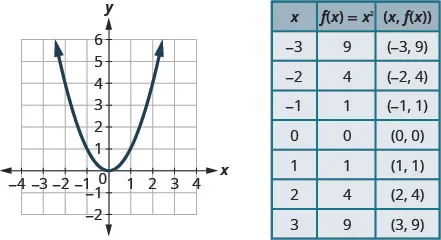Every quadratic function has a graph that looks like this. We call this figure a parabola.

Let’s practice graphing a parabola by plotting a few points.

### Example 9.42

Graph $f(x)=x2−1.f(x)=x2−1.$

### Try It 9.83

Graph $f(x)=−x2.f(x)=−x2.$.

### Try It 9.84

Graph $f(x)=x2+1.f(x)=x2+1.$

All graphs of quadratic functions of the form f (x) = ax2 + bx + c are parabolas that open upward or downward. See Figure 9.2.

Figure 9.2

Notice that the only difference in the two functions is the negative sign before the quadratic term (x2 in the equation of the graph in Figure 9.2). When the quadratic term, is positive, the parabola opens upward, and when the quadratic term is negative, the parabola opens downward.

### Parabola Orientation

For the graph of the quadratic function f (x) = ax2 + bx + c, if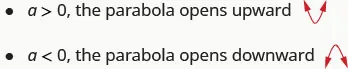### Example 9.43

Determine whether each parabola opens upward or downward:

$f(x)=−3x2+2x−4f(x)=−3x2+2x−4$ $f(x)=6x2+7x−9.f(x)=6x2+7x−9.$

### Try It 9.85

Determine whether the graph of each function is a parabola that opens upward or downward:

$f(x)=2x2+5x−2f(x)=2x2+5x−2$ $f(x)=−3x2−4x+7.f(x)=−3x2−4x+7.$

### Try It 9.86

Determine whether the graph of each function is a parabola that opens upward or downward:

$f(x)=−2x2−2x−3f(x)=−2x2−2x−3$ $f(x)=5x2−2x−1.f(x)=5x2−2x−1.$

### Find the Axis of Symmetry and Vertex of a Parabola

Look again at Figure 9.2. Do you see that we could fold each parabola in half and then one side would lie on top of the other? The ‘fold line’ is a line of symmetry. We call it the axis of symmetry of the parabola.

We show the same two graphs again with the axis of symmetry. See Figure 9.3.

Figure 9.3

The equation of the axis of symmetry can be derived by using the Quadratic Formula. We will omit the derivation here and proceed directly to using the result. The equation of the axis of symmetry of the graph of f (x) = ax2 + bx + c is $x=−b2a.x=−b2a.$

So to find the equation of symmetry of each of the parabolas we graphed above, we will substitute into the formula $x=−b2a.x=−b2a.$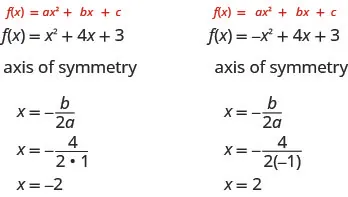Notice that these are the equations of the dashed blue lines on the graphs.

The point on the parabola that is the lowest (parabola opens up), or the highest (parabola opens down), lies on the axis of symmetry. This point is called the vertex of the parabola.

We can easily find the coordinates of the vertex, because we know it is on the axis of symmetry. This means its
x-coordinate is $−b2a.−b2a.$ To find the y-coordinate of the vertex we substitute the value of the x-coordinate into the quadratic function.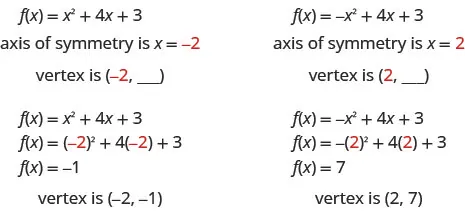### Axis of Symmetry and Vertex of a Parabola

The graph of the function f (x) = ax2 + bx + c is a parabola where:

• the axis of symmetry is the vertical line $x=−b2a.x=−b2a.$
• the vertex is a point on the axis of symmetry, so its x-coordinate is $−b2a.−b2a.$
• the y-coordinate of the vertex is found by substituting $x=−b2ax=−b2a$ into the quadratic equation.

### Example 9.44

For the graph of $f(x)=3x2−6x+2f(x)=3x2−6x+2$ find:

the axis of symmetry the vertex.

### Try It 9.87

For the graph of $f(x)=2x2−8x+1f(x)=2x2−8x+1$ find:

the axis of symmetry the vertex.

### Try It 9.88

For the graph of $f(x)=2x2−4x−3f(x)=2x2−4x−3$ find:

the axis of symmetry the vertex.

### Find the Intercepts of a Parabola

When we graphed linear equations, we often used the x- and y-intercepts to help us graph the lines. Finding the coordinates of the intercepts will help us to graph parabolas, too.

Remember, at the y-intercept the value of x is zero. So to find the y-intercept, we substitute x = 0 into the function.

Let’s find the y-intercepts of the two parabolas shown in Figure 9.4.

Figure 9.4

An x-intercept results when the value of f (x) is zero. To find an x-intercept, we let f (x) = 0. In other words, we will need to solve the equation 0 = ax2 + bx + c for x.

$f(x)=ax2+bx+c0=ax2+bx+cf(x)=ax2+bx+c0=ax2+bx+c$

Solving quadratic equations like this is exactly what we have done earlier in this chapter!

We can now find the x-intercepts of the two parabolas we looked at. First we will find the x-intercepts of the parabola whose function is f (x) = x2 + 4x + 3.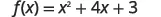Let $f(x)=0f(x)=0$.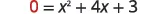Factor.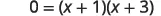Use the Zero Product Property.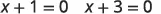Solve.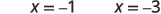The x-intercepts are $(−1,0)(−1,0)$ and $(−3,0)(−3,0)$.

Now we will find the x-intercepts of the parabola whose function is f (x) = −x2 + 4x + 3.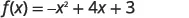Let $f(x)=0f(x)=0$.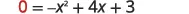This quadratic does not factor, sowe use the Quadratic Formula.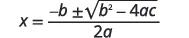$a=−1,b=4,c=3a=−1,b=4,c=3$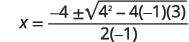Simplify.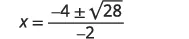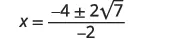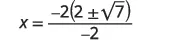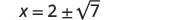The x-intercepts are $(2+7,0)(2+7,0)$ and$(2−7,0)(2−7,0)$.

We will use the decimal approximations of the x-intercepts, so that we can locate these points on the graph,

$(2+7,0)≈(4.6,0)(2−7,0)≈(−0.6,0)(2+7,0)≈(4.6,0)(2−7,0)≈(−0.6,0)$

Do these results agree with our graphs? See Figure 9.5.

Figure 9.5

### Find the Intercepts of a Parabola

To find the intercepts of a parabola whose function is $f(x)=ax2+bx+c:f(x)=ax2+bx+c:$

$y-interceptx-interceptsLetx=0and solve forf(x).Letf(x)=0and solve forx.y-interceptx-interceptsLetx=0and solve forf(x).Letf(x)=0and solve forx.$

### Example 9.45

Find the intercepts of the parabola whose function is $f(x)=x2−2x−8.f(x)=x2−2x−8.$

### Try It 9.89

Find the intercepts of the parabola whose function is $f(x)=x2+2x−8.f(x)=x2+2x−8.$

### Try It 9.90

Find the intercepts of the parabola whose function is $f(x)=x2−4x−12.f(x)=x2−4x−12.$

In this chapter, we have been solving quadratic equations of the form ax2 + bx + c = 0. We solved for x and the results were the solutions to the equation.

We are now looking at quadratic functions of the form f (x) = ax2 + bx + c. The graphs of these functions are parabolas. The x-intercepts of the parabolas occur where f (x) = 0.

For example:

$Quadratic equationQuadratic function x2−2x−15=0(x−5)(x+3)=0x−5=0x+3=0x=5x=−3Letf(x)=0.f(x)=x2−2x−150=x2−2x−150=(x−5)(x+3)x−5=0x+3=0x=5x=−3(5,0)and(−3,0)x-interceptsQuadratic equationQuadratic function x2−2x−15=0(x−5)(x+3)=0x−5=0x+3=0x=5x=−3Letf(x)=0.f(x)=x2−2x−150=x2−2x−150=(x−5)(x+3)x−5=0x+3=0x=5x=−3(5,0)and(−3,0)x-intercepts$

The solutions of the quadratic function are the x values of the x-intercepts.

Earlier, we saw that quadratic equations have 2, 1, or 0 solutions. The graphs below show examples of parabolas for these three cases. Since the solutions of the functions give the x-intercepts of the graphs, the number of x-intercepts is the same as the number of solutions.

Previously, we used the discriminant to determine the number of solutions of a quadratic function of the form $ax2+bx+c=0.ax2+bx+c=0.$ Now we can use the discriminant to tell us how many x-intercepts there are on the graph.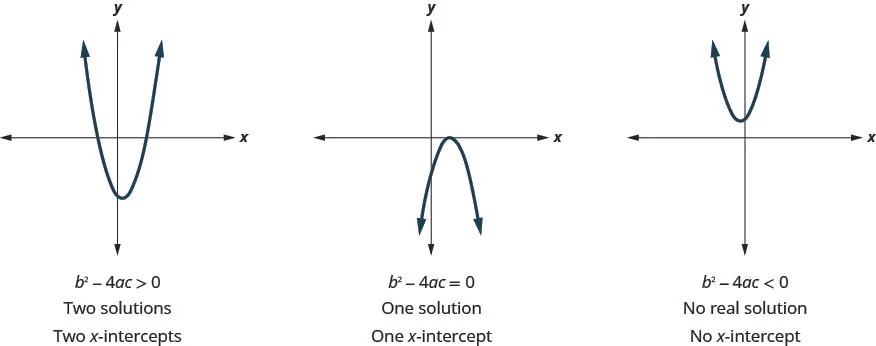Before you to find the values of the x-intercepts, you may want to evaluate the discriminant so you know how many solutions to expect.

### Example 9.46

Find the intercepts of the parabola for the function $f(x)=5x2+x+4.f(x)=5x2+x+4.$

### Try It 9.91

Find the intercepts of the parabola whose function is $f(x)=3x2+4x+4.f(x)=3x2+4x+4.$

### Try It 9.92

Find the intercepts of the parabola whose function is $f(x)=x2−4x−5.f(x)=x2−4x−5.$

### Graph Quadratic Functions Using Properties

Now we have all the pieces we need in order to graph a quadratic function. We just need to put them together. In the next example we will see how to do this.

### Example 9.47

#### How to Graph a Quadratic Function Using Properties

Graph f (x) = x2 −6x + 8 by using its properties.

### Try It 9.93

Graph f (x) = x2 + 2x − 8 by using its properties.

### Try It 9.94

Graph f (x) = x2 − 8x + 12 by using its properties.

We list the steps to take in order to graph a quadratic function here.

### How To

#### To graph a quadratic function using properties.

1. Step 1. Determine whether the parabola opens upward or downward.
2. Step 2. Find the equation of the axis of symmetry.
3. Step 3. Find the vertex.
4. Step 4. Find the y-intercept. Find the point symmetric to the y-intercept across the axis of symmetry.
5. Step 5. Find the x-intercepts. Find additional points if needed.
6. Step 6. Graph the parabola.

We were able to find the x-intercepts in the last example by factoring. We find the x-intercepts in the next example by factoring, too.

### Example 9.48

Graph f (x) = –x2 + 6x − 9 by using its properties.

### Try It 9.95

Graph f (x) = −3x2 + 12x − 12 by using its properties.

### Try It 9.96

Graph f (x) = 4x2 + 24x + 36 by using its properties.

For the graph of f (x) = −x2 + 6x − 9, the vertex and the x-intercept were the same point. Remember how the discriminant determines the number of solutions of a quadratic equation? The discriminant of the equation 0 = −x2 + 6x − 9 is 0, so there is only one solution. That means there is only one x-intercept, and it is the vertex of the parabola.

How many x-intercepts would you expect to see on the graph of f (x) = x2 + 4x + 5?

### Example 9.49

Graph f (x) = x2 + 4x + 5 by using its properties.

### Try It 9.97

Graph f (x) = x2 − 2x + 3 by using its properties.

### Try It 9.98

Graph f (x) = −3x2 − 6x − 4 by using its properties.

Finding the y-intercept by finding f (0) is easy, isn’t it? Sometimes we need to use the Quadratic Formula to find the x-intercepts.

### Example 9.50

Graph f (x) = 2x2 − 4x − 3 by using its properties.

### Try It 9.99

Graph f (x) = 5x2 + 10x + 3 by using its properties.

### Try It 9.100

Graph f (x) = −3x2 − 6x + 5 by using its properties.

### Solve Maximum and Minimum Applications

Knowing that the vertex of a parabola is the lowest or highest point of the parabola gives us an easy way to determine the minimum or maximum value of a quadratic function. The y-coordinate of the vertex is the minimum value of a parabola that opens upward. It is the maximum value of a parabola that opens downward. See Figure 9.6.

Figure 9.6

### Minimum or Maximum Values of a Quadratic Function

The y-coordinate of the vertex of the graph of a quadratic function is the

• minimum value of the quadratic equation if the parabola opens upward.
• maximum value of the quadratic equation if the parabola opens downward.

### Example 9.51

Find the minimum or maximum value of the quadratic function $f(x)=x2+2x−8.f(x)=x2+2x−8.$

### Try It 9.101

Find the maximum or minimum value of the quadratic function $f(x)=x2−8x+12.f(x)=x2−8x+12.$

### Try It 9.102

Find the maximum or minimum value of the quadratic function $f(x)=−4x2+16x−11.f(x)=−4x2+16x−11.$

We have used the formula

$h(t)=−16t2+v0t+h0h(t)=−16t2+v0t+h0$

to calculate the height in feet, h , of an object shot upwards into the air with initial velocity, v0, after t seconds .

This formula is a quadratic function, so its graph is a parabola. By solving for the coordinates of the vertex (t, h), we can find how long it will take the object to reach its maximum height. Then we can calculate the maximum height.

### Example 9.52

The quadratic equation h(t) = −16t2 + 176t + 4 models the height of a volleyball hit straight upwards with velocity 176 feet per second from a height of 4 feet.

How many seconds will it take the volleyball to reach its maximum height? Find the maximum height of the volleyball.

### Try It 9.103

Solve, rounding answers to the nearest tenth.

The quadratic function h(t) = −16t2 + 128t + 32 is used to find the height of a stone thrown upward from a height of 32 feet at a rate of 128 ft/sec. How long will it take for the stone to reach its maximum height? What is the maximum height?

### Try It 9.104

A path of a toy rocket thrown upward from the ground at a rate of 208 ft/sec is modeled by the quadratic function of h(t) = −16t2 + 208t. When will the rocket reach its maximum height? What will be the maximum height?

### Media

Access these online resources for additional instruction and practice with graphing quadratic functions using properties.

### Section 9.6 Exercises

#### Practice Makes Perfect

Recognize the Graph of a Quadratic Function

In the following exercises, graph the functions by plotting points.

229.

$f ( x ) = x 2 + 3 f ( x ) = x 2 + 3$

230.

$f ( x ) = x 2 − 3 f ( x ) = x 2 − 3$

231.

$y = − x 2 + 1 y = − x 2 + 1$

232.

$f ( x ) = − x 2 − 1 f ( x ) = − x 2 − 1$

For each of the following exercises, determine if the parabola opens up or down.

233.

$f(x)=−2x2−6x−7f(x)=−2x2−6x−7$ $f(x)=6x2+2x+3f(x)=6x2+2x+3$

234.

$f(x)=4x2+x−4f(x)=4x2+x−4$ $f(x)=−9x2−24x−16f(x)=−9x2−24x−16$

235.

$f(x)=−3x2+5x−1f(x)=−3x2+5x−1$ $f(x)=2x2−4x+5f(x)=2x2−4x+5$

236.

$f(x)=x2+3x−4f(x)=x2+3x−4$ $f(x)=−4x2−12x−9f(x)=−4x2−12x−9$

Find the Axis of Symmetry and Vertex of a Parabola

In the following functions, find the equation of the axis of symmetry and the vertex of its graph.

237.

$f ( x ) = x 2 + 8 x − 1 f ( x ) = x 2 + 8 x − 1$

238.

$f ( x ) = x 2 + 10 x + 25 f ( x ) = x 2 + 10 x + 25$

239.

$f ( x ) = − x 2 + 2 x + 5 f ( x ) = − x 2 + 2 x + 5$

240.

$f ( x ) = −2 x 2 − 8 x − 3 f ( x ) = −2 x 2 − 8 x − 3$

Find the Intercepts of a Parabola

In the following exercises, find the intercepts of the parabola whose function is given.

241.

$f ( x ) = x 2 + 7 x + 6 f ( x ) = x 2 + 7 x + 6$

242.

$f ( x ) = x 2 + 10 x − 11 f ( x ) = x 2 + 10 x − 11$

243.

$f ( x ) = x 2 + 8 x + 12 f ( x ) = x 2 + 8 x + 12$

244.

$f ( x ) = x 2 + 5 x + 6 f ( x ) = x 2 + 5 x + 6$

245.

$f ( x ) = − x 2 + 8 x − 19 f ( x ) = − x 2 + 8 x − 19$

246.

$f ( x ) = −3 x 2 + x − 1 f ( x ) = −3 x 2 + x − 1$

247.

$f ( x ) = x 2 + 6 x + 13 f ( x ) = x 2 + 6 x + 13$

248.

$f ( x ) = x 2 + 8 x + 12 f ( x ) = x 2 + 8 x + 12$

249.

$f ( x ) = 4 x 2 − 20 x + 25 f ( x ) = 4 x 2 − 20 x + 25$

250.

$f ( x ) = − x 2 − 14 x − 49 f ( x ) = − x 2 − 14 x − 49$

251.

$f ( x ) = − x 2 − 6 x − 9 f ( x ) = − x 2 − 6 x − 9$

252.

$f ( x ) = 4 x 2 + 4 x + 1 f ( x ) = 4 x 2 + 4 x + 1$

In the following exercises, graph the function by using its properties.

253.

$f ( x ) = x 2 + 6 x + 5 f ( x ) = x 2 + 6 x + 5$

254.

$f ( x ) = x 2 + 4 x − 12 f ( x ) = x 2 + 4 x − 12$

255.

$f ( x ) = x 2 + 4 x + 3 f ( x ) = x 2 + 4 x + 3$

256.

$f ( x ) = x 2 − 6 x + 8 f ( x ) = x 2 − 6 x + 8$

257.

$f ( x ) = 9 x 2 + 12 x + 4 f ( x ) = 9 x 2 + 12 x + 4$

258.

$f ( x ) = − x 2 + 8 x − 16 f ( x ) = − x 2 + 8 x − 16$

259.

$f ( x ) = − x 2 + 2 x − 7 f ( x ) = − x 2 + 2 x − 7$

260.

$f ( x ) = 5 x 2 + 2 f ( x ) = 5 x 2 + 2$

261.

$f ( x ) = 2 x 2 − 4 x + 1 f ( x ) = 2 x 2 − 4 x + 1$

262.

$f ( x ) = 3 x 2 − 6 x − 1 f ( x ) = 3 x 2 − 6 x − 1$

263.

$f ( x ) = 2 x 2 − 4 x + 2 f ( x ) = 2 x 2 − 4 x + 2$

264.

$f ( x ) = −4 x 2 − 6 x − 2 f ( x ) = −4 x 2 − 6 x − 2$

265.

$f ( x ) = − x 2 − 4 x + 2 f ( x ) = − x 2 − 4 x + 2$

266.

$f ( x ) = x 2 + 6 x + 8 f ( x ) = x 2 + 6 x + 8$

267.

$f ( x ) = 5 x 2 − 10 x + 8 f ( x ) = 5 x 2 − 10 x + 8$

268.

$f ( x ) = −16 x 2 + 24 x − 9 f ( x ) = −16 x 2 + 24 x − 9$

269.

$f ( x ) = 3 x 2 + 18 x + 20 f ( x ) = 3 x 2 + 18 x + 20$

270.

$f ( x ) = −2 x 2 + 8 x − 10 f ( x ) = −2 x 2 + 8 x − 10$

Solve Maximum and Minimum Applications

In the following exercises, find the maximum or minimum value of each function.

271.

$f ( x ) = 2 x 2 + x − 1 f ( x ) = 2 x 2 + x − 1$

272.

$y = −4 x 2 + 12 x − 5 y = −4 x 2 + 12 x − 5$

273.

$y = x 2 − 6 x + 15 y = x 2 − 6 x + 15$

274.

$y = − x 2 + 4 x − 5 y = − x 2 + 4 x − 5$

275.

$y = −9 x 2 + 16 y = −9 x 2 + 16$

276.

$y = 4 x 2 − 49 y = 4 x 2 − 49$

In the following exercises, solve. Round answers to the nearest tenth.

277.

An arrow is shot vertically upward from a platform 45 feet high at a rate of 168 ft/sec. Use the quadratic function h(t) = −16t2 + 168t + 45 find how long it will take the arrow to reach its maximum height, and then find the maximum height.

278.

A stone is thrown vertically upward from a platform that is 20 feet height at a rate of 160 ft/sec. Use the quadratic function h(t) = −16t2 + 160t + 20 to find how long it will take the stone to reach its maximum height, and then find the maximum height.

279.

A ball is thrown vertically upward from the ground with an initial velocity of 109 ft/sec. Use the quadratic function h(t) = −16t2 + 109t + 0 to find how long it will take for the ball to reach its maximum height, and then find the maximum height.

280.

A ball is thrown vertically upward from the ground with an initial velocity of 122 ft/sec. Use the quadratic function h(t) = −16t2 + 122t + 0 to find how long it will take for the ball to reach its maximum height, and then find the maximum height.

281.

A computer store owner estimates that by charging x dollars each for a certain computer, he can sell 40 − x computers each week. The quadratic function R(x) = −x2 +40x is used to find the revenue, R, received when the selling price of a computer is x, Find the selling price that will give him the maximum revenue, and then find the amount of the maximum revenue.

282.

A retailer who sells backpacks estimates that by selling them for x dollars each, he will be able to sell 100 − x backpacks a month. The quadratic function R(x) = −x2 +100x is used to find the R, received when the selling price of a backpack is x. Find the selling price that will give him the maximum revenue, and then find the amount of the maximum revenue.

283.

A retailer who sells fashion boots estimates that by selling them for x dollars each, he will be able to sell 70 − x boots a week. Use the quadratic function R(x) = −x2 +70x to find the revenue received when the average selling price of a pair of fashion boots is x. Find the selling price that will give him the maximum revenue, and then find the amount of the maximum revenue per day.

284.

A cell phone company estimates that by charging x dollars each for a certain cell phone, they can sell 8 − x cell phones per day. Use the quadratic function R(x) = −x2 +8x to find the revenue received per day when the selling price of a cell phone is x. Find the selling price that will give them the maximum revenue per day, and then find the amount of the maximum revenue.

285.

A rancher is going to fence three sides of a corral next to a river. He needs to maximize the corral area using 240 feet of fencing. The quadratic equation $A(x)=x120−x2A(x)=x120−x2$ gives the area of the corral, A, for the length, x, of the corral along the river. Find the length of the corral along the river that will give the maximum area, and then find the maximum area of the corral.

286.

A veterinarian is enclosing a rectangular outdoor running area against his building for the dogs he cares for. He needs to maximize the area using 100 feet of fencing. The quadratic function $A(x)=x50−x2A(x)=x50−x2$ gives the area, A, of the dog run for the length, x, of the building that will border the dog run. Find the length of the building that should border the dog run to give the maximum area, and then find the maximum area of the dog run.

287.

A land owner is planning to build a fenced in rectangular patio behind his garage, using his garage as one of the “walls.” He wants to maximize the area using 80 feet of fencing. The quadratic function A(x) = x(80 − 2x) gives the area of the patio, where x is the width of one side. Find the maximum area of the patio.

288.

A family of three young children just moved into a house with a yard that is not fenced in. The previous owner gave them 300 feet of fencing to use to enclose part of their backyard. Use the quadratic function $A(x)=x150−x2A(x)=x150−x2$ determine the maximum area of the fenced in yard.

#### Writing Exercise

289.

How do the graphs of the functions $f(x)=x2f(x)=x2$ and $f(x)=x2−1f(x)=x2−1$ differ? We graphed them at the start of this section. What is the difference between their graphs? How are their graphs the same?

290.

Explain the process of finding the vertex of a parabola.

291.

Explain how to find the intercepts of a parabola.

292.

How can you use the discriminant when you are graphing a quadratic function?

#### Self Check

After completing the exercises, use this checklist to evaluate your mastery of the objectives of this section.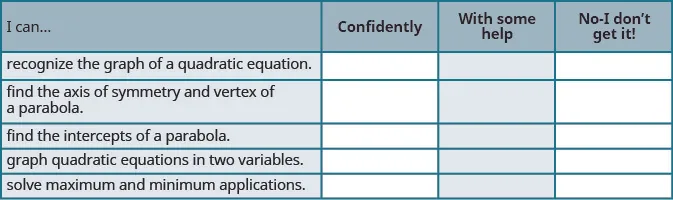After looking at the checklist, do you think you are well-prepared for the next section? Why or why not?

Order a print copy

As an Amazon Associate we earn from qualifying purchases.